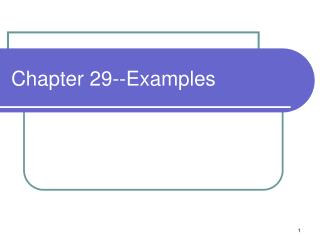DownloadDownload PresentationChapter 29--Examples

# Chapter 29--Examples

Download Presentation## Chapter 29--Examples

- - - - - - - - - - - - - - - - - - - - - - - - - - - E N D - - - - - - - - - - - - - - - - - - - - - - - - - - -
##### Presentation Transcript

1. Chapter 29--Examples

2. Problem • Derive the equation relating the total charge Q that flows through a search coil (Conceptual Example 29.3 in Section 29.2) to the magnetic field B. The search coil has N turns, each with area A and the flux through the coil is decreased from its initial value to zero in a time Dt. The resistance of the coil is R and the total charge is Q=I Dt where I is the average current induced by the change in flux

3. From Example 29.3 • A search coil is a practical way to measure magnetic field strength. It uses a small, closely wound coil with N turns. The coil, of area A, is initially held so that its area vector A is aligned with a magnetic field with magnitude B. The coil is quickly pulled out of the field or rotated. • Initially the flux through the field is F=NBA. When it leaves the field or rotated, F goes to zero. As F decreases, there is a momentary induced current which is measured. The amount of current is proportional to the field strength.

4. Solution • EMF=-DF/Dt • DF=F2–F1 • Where F1 =NBA and F2 =0 • DF=-NBA • EMF=NBA/Dt • V=iR where V=EMF • EMF=NBA/Dt=iR • i=NBA/RDt • Q=iDt=NBA/R

5. Part B • In a credit card reader, the magnetic strip on the back of the card is “swiped” past a coil within the reader. Explain using the ideas of the search coil how the reader can decode information stored in the pattern of magnetization in the strip.

6. Solution • The card reader contains a search coil. • The search coil produces high EMF and low EMF as the card is swiped. • A high EMF is treated as a binary 1 and a low EMF is treated as a binary 0 • Ascii information (character codes between 1 and 64) can be stored there in a few bits (6-bits or 26).

7. Problem A circular loop of flexible iron wire has an initial circumference of 165 cm but its circumference is decreasing at a rate of 12 cm/s due to a tangential pull on the wire. The loop is in a constant, uniform magnetic field oriented perpendicular to the plane of the loop and with a magnitude of 0.5 T • Find the EMF induced in the loop at the instant when 9 s have passed. • Find the direction of the current in the loop as viewed looking in the direction of the magnetic field.

8. EMF=-DF/Dt=-B*DA/Dt • F=BA • dF/dt=BdA/dt • c=2pr so A=pr2 • r=c/2p so A=c2/4p • dA/dt=d/dt(c2/4p)= (2c/4p)dc/dt • dF/dt= B(c/2p)dc/dt • Where B=0.5 • dc/dt=0.12 m/s • At 9s, c=1.65-.12*(9s)=0.57 m • dF/dt=5.44 x 10-3 V • Since F is decreasing, the EMF is positive. Since EMF positive, point thumb along A and look at fingers. • They curl counterclockwise.

9. Problem Suppose the loop in the figure below is • Rotated about the y-axis • Rotated about the x-axis • Rotated about an edge parallel to the z-axis. What is the maximum induced EMF in each case if A=600 cm2, w=35 rad/s, and B=.45T?

10. Case a—Rotating about y-axis

11. Case b—rotating about the x-axis • The normal to the surface is in the same direction as B during this rotation. Thus BA=constant • d/dt(constant)=0 so EMF=0

12. Case c—Rotating about z-axis

13. Problem The figure below shows two parallel loops of wire having a common axis. The smaller loop (radius r) is above the larger loop (radius R) by a distance x>>R. Consequently, the magnetic field due to current i in the larger loop is nearly constant throughout the smaller loop. Suppose that x is increasing at a constant rate, v. • Determine the magnetic flux though area of the smaller loop as a function of x. • In the smaller loop find • The induced EMF • The direction of the induced current. Radius=r Radius=R x i

14. Magnetic Field of a Circular Loop By the RH Rule, the field is upward in the small ring

15. EMF in smaller loop

16. Direction of current • Assume that normal to smaller loop is positive upwards • B, then, is in positive direction • But F is decreasing or has a negative d dF /dt • A negative * negative=positive so EMF is + • RH Thumb in up direction, fingers curl counter clockwise!